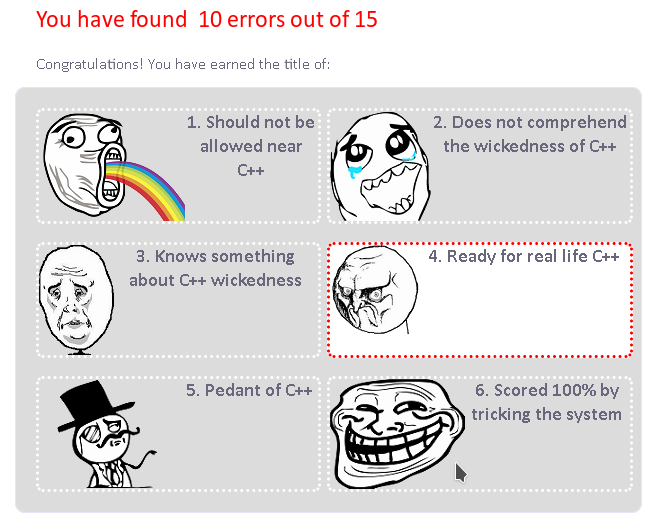# 16:9 iframe

While updating YouTube embeds today, I also updated embed styling. I used this insanity taken from I don’t even remember where:

``````.videoblock > .content {
overflow: hidden;
position: relative;

> iframe {
border: 0;
position: absolute;
height: 100%;
width: 100%;
top: 0;
left: 0;
}
}``````

Much simpler now:

``````.videoblock > .content > iframe {
width: 100%;
height: 100%;
aspect-ratio: 16 / 9;
}``````

Almost a year ago I scrapped my WordPress and started moving my blog to Pelican, and now it’s finally ready. Blog has been transferred and a new template has been developed, Tumblr inspired, UIkit powered, hand crafted, with dark mode using `prefers-color-scheme: dark`. Right for the next Christmas.

# C++ Quiz

A rather hardcore C++ quiz from PVS Studio developers: q.viva64.com# Almost Monkey Patching in С++

```include <string>

std::string operator*(std::string& s, unsigned int n)
{
std::string cml;

cml.reserve(s.size() * n);

for (decltype(n) i = 0; i < n; i++) {
cml += s;
}

return cml;
}

int main()
{
std::string s   = "test";
std::string sss = s*3;

std::cout << sss << std::endl; // testtesttest
}
```

# PHP 5.6 — variadic func / splat operator

```<?php

function func(\$a, \$b, ...\$params)
{
var_dump(\$a, \$b, \$params);
}

func(1,2,3,4,5);
// int(1)
// int(2)
// array(3) {
//   =>
//   int(3)
//  =>
//  int(4)
//  =>
//  int(5)
// }
// cool!

\$a = [1,2,3,4,5];

func(...\$a);
// int(1)
// int(2)
// array(3) {
//   =>
//   int(3)
//  =>
//  int(4)
//  =>
//  int(5)
// }
// even cooler!

\$a['qq'] = 'qq';

func(...\$a);
// PHP Catchable fatal error:
// Cannot unpack array with string keys in /tmp/test.php on line 16
// okay :(

\$b = [];
\$b = 1;
\$b = 4;
\$b = 2;
\$b = 5;
\$b = 3;

func(...\$b);
// int(1)
// int(4)
// array(3) {
//   =>
//   int(2)
//   =>
//   int(5)
//   =>
//   int(3)
// }
// WAT?
```

# Am I completely lost to society?```#!/usr/bin/ruby

require 'date'

birth = Date.parse(gets, true)
today = Date.today
age   = today-birth # in days

min_age = (2 * (age - 7 * 365.25)).round
max_age = (age/2 + 7 * 365.25).round

min_birth = Date.today - min_age
max_birth = Date.today - max_age

puts "Your age pool is #{min_birth.to_s} - #{max_birth.to_s}"

puts "You're too young ^__^" if min_birth > max_birth
```